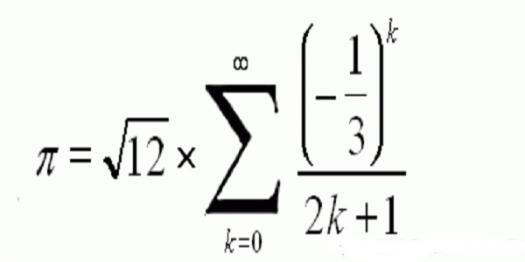# Do You Know Madhava Series?

Approved & Edited by ProProfs Editorial Team
The editorial team at ProProfs Quizzes consists of a select group of subject experts, trivia writers, and quiz masters who have authored over 10,000 quizzes taken by more than 100 million users. This team includes our in-house seasoned quiz moderators and subject matter experts. Our editorial experts, spread across the world, are rigorously trained using our comprehensive guidelines to ensure that you receive the highest quality quizzes.
| By Zlatan Aleksic
Z
Zlatan Aleksic
Community Contributor
Quizzes Created: 206 | Total Attempts: 1,002,931
Questions: 10 | Attempts: 100SettingsMadhava series are product of Madhava of Sangamagrama's research and his contribution to mathematics. Your knowledge will be challenged with this quiz and you will find out are you an expert on this topic!

• 1.

### Where from is Madhava of Sangamagrama?

• A.

Sangamagrama

• B.

Mira-Bhayandar

• C.

Bareily

• D.

Bhiwandi

A. Sangamagrama
Explanation
Madhava of Sangamagrama is from Sangamagrama.

Rate this question:

• 2.

• A.

• B.

• C.

• D.

• 3.

### What is the number of Pi?

• A.

3.14180

• B.

3.15159

• C.

3.14159

• D.

3.14286

C. 3.14159
Explanation
The number of Pi is a mathematical constant that represents the ratio of a circle's circumference to its diameter. It is approximately equal to 3.14159.

Rate this question:

• 4.

### Madhava obtained a value for Pi with how many decimal places?

• A.

10

• B.

11

• C.

12

• D.

13

D. 13
Explanation
Madhava obtained a value for Pi with 13 decimal places. This means that he calculated Pi accurately up to the thirteenth decimal place.

Rate this question:

• 5.

• A.

Accurate table of sines

• B.

Astrology chart

• C.

Periodic system of elements

• D.

Composition ring

A. Accurate table of sines
Explanation
Madhava has composed an accurate table of sines. This means that he has created a comprehensive and precise chart that displays the values of the sine function for various angles. Such a table would be useful in various mathematical and scientific calculations involving trigonometry, enabling accurate calculations and measurements in fields such as physics, engineering, and astronomy.

Rate this question:

• 6.

### How many of Madhava's works, containing any of the series expressions attributed to him, have survived?

• A.

3

• B.

0

• C.

104

• D.

26

B. 0
Explanation
None of Madhava's works containing any of the series expressions attributed to him have survived.

Rate this question:

• 7.

### Who has rediscovered Madhava series?

• A.

Pierre de Fermat

• B.

Leonhard Euler

• C.

James Gregory

• D.

Rene Descartes

C. James Gregory
Explanation
James Gregory is the correct answer because he is the one who rediscovered the Madhava series. The Madhava series, also known as the Kerala school series, is a mathematical series that was originally developed by the Indian mathematician Madhava of Sangamagrama in the 14th century. However, the series was lost and forgotten over time. It was James Gregory, a Scottish mathematician, who rediscovered and brought attention to the series in the 17th century. Gregory's rediscovery of the Madhava series played a crucial role in the development of calculus and the expansion of mathematical knowledge.

Rate this question:

• 8.

### When did James Gregory rediscovered Madhava series?

• A.

1793

• B.

1723

• C.

1650

• D.

1668

D. 1668
Explanation
James Gregory rediscovered Madhava series in 1668.

Rate this question:

• 9.

### For which study did Madhava laid the foundations?

• A.

Calculus

• B.

Geometry

• C.

Algebra

• D.

None of the above

A. Calculus
Explanation
Madhava laid the foundations for the study of Calculus. Calculus is a branch of mathematics that deals with the study of change and motion. It includes concepts such as limits, derivatives, and integrals. Madhava, an Indian mathematician and astronomer, made significant contributions to the development of Calculus in the 14th century. He is known for his work on infinite series and the discovery of the power series representation of trigonometric functions. Madhava's contributions laid the groundwork for the later development of Calculus by European mathematicians such as Isaac Newton and Gottfried Wilhelm Leibniz.

Rate this question:

• 10.

### In which century was Madhava born?

• A.

13th century

• B.

14th century

• C.

15th century

• D.

12th century